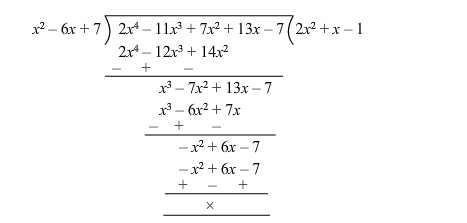# Find all the zeros of the polynomialQuestion:

Find all the zeros of the polynomial $\left(2 x^{4}-11 x^{3}+7 x^{2}+13 x\right)$, it being given that two if its zeros are $3+\sqrt{2}$ and $3-\sqrt{2}$.

Solution:

The given polynomial is $f(x)=2 x^{4}-11 x^{3}+7 x^{2}+13 x-7$.

Since $(3+\sqrt{2})$ and $(3-\sqrt{2})$ are the zeroes of $f(x)$, it follows that each one of $(x+3+\sqrt{2})$ and $(x+3-\sqrt{2})$ is a factor of $f(x)$.

Consequently, $[x-(3+\sqrt{2})][x-(3-\sqrt{2})]=[(x-3)-\sqrt{2}][(x-3)+\sqrt{2}]$

$=\left[(x-3)^{2}-2\right]=x^{2}-6 x+7$, which is a factor of $f(x)$

On dividing $f(x)$ by $\left(x^{2}-6 x+7\right)$, we get:$\therefore f(x)=0$

$\Rightarrow 2 x^{4}-11 x^{3}+7 x^{2}+13 x-7=0$

$=>\left(x^{2}-6 x+7\right)\left(2 x^{2}+x-1\right)=0$

$=>(x+3+\sqrt{2})(x+3-\sqrt{2})(2 x-1)(x+1)=0$

$=>x=-3-\sqrt{2}$ or $x=-3+\sqrt{2}$ or $x=\frac{1}{2}$ or $x=-1$

Hence, all the zeros are $(-3-\sqrt{2}),(-3+\sqrt{2}), \frac{1}{2}$ and $-1$.